Neural Networks:MLP Non-linear Transfer Function

The formula, look-up table, linear interpolation, new version of Sigmoid(), prototyping in QuickBASIC, the 'C' version, SigGen()'s coding, Sigmoid()'s coding, test results, Full Source Listing.

The Sigmoid Function

The sigmoid function is so-called because it is shaped like one form of the Greek letter Sigma. Its purpose, within an artificial neuron, is to generate a degree of non-linearity between the neuron's input and output.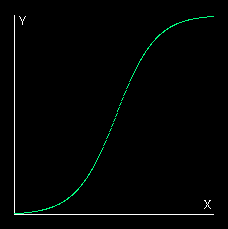The adjacent applet creates a sigmoid curve by it­er­ating the difference equation y += k(1 − y). This operates over the range 0 to +1 for both input and output. However, perhaps the most familiar way of creating a sigmoid curve is with the natural expon­ent­ial function: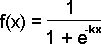If you are using Microsoft Windows and a Security Warning box pops up saying that the application has been blocked from running because it is "untrusted" please click here. If you get a warning message with Linux, please click here.

The basic formula for the sigmoid function above caters only for 'real' values of x from −1 to +1 and produces an output f(x) over the range 0 to +1.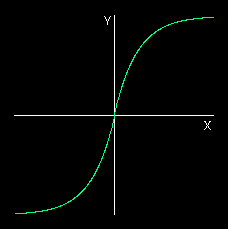The enhanced version below provides a bipolar out­put over the same range as the input.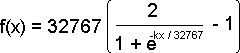This version of the formula also rescales the input and the output to a range of −32767 to +32767 which is the range of the 16-bit integer native to the machine used on this project.

This formula can be written as a 'C' statement as follows: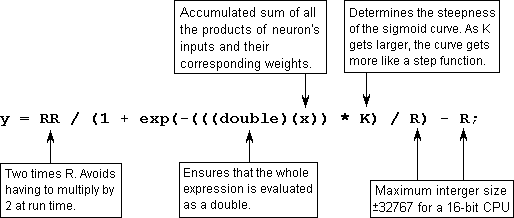Since we need only 16-bit precision in the output, y can be a 16-bit int. The exp() function takes a floating point double argument, and would therefore force the result of the multiplication and division to a floating point double. However, in order to preserve the 16-bit precision of the input through the calculation, we must cast x to a floating point double before multiplying it by K and subsequently dividing it by R.

We shall now build this formula into a 'C' function we shall call Sigmoid().

int Sigmoid(int x) {
#define R  32767   //max value of a signed 16-bit interger
#define RR 65534   //double it to avoid run-time doubling
#define K      8   //suitable value for K
return(RR / (1 + exp( -(((double)(x)) * K) / R) - R);
}

Unfortunately, this function was found to take 5·4 milliseconds to execute on an 80286 PC. Since it is at the very time-critical centre of a network, this is unaccept­ably slow. A very small increase in speed is possible by placing the formula directly into the neural network function as a single statement, but the gain is almost imperceptible. A better solution is vital.

Look-up Table

A vast speed improvement would obviously be achieved if all 32768 plots covering the range of the 16-bit input and output values were placed in a look-up table. However this would require 64k of memory to hold the table which is also un­ac­ceptable. A compromise is to have a smaller table and use linear interpolation to find values of f(x) for values of x in between two of the spot values of x for which table entries have been made.

Linear Interpolation

This works on the bold assumption that any section of the sigmoid curve, between two successive tabulated plots, is a straight line. We know it is not, but we hope that the real curve does not bend too far away from our straight line and thereby keep the error within acceptable limits. Assuming the line is straight enough, we can find the value of f(x) corresponding to an inter-plot value of x by simple geo­metry as follows: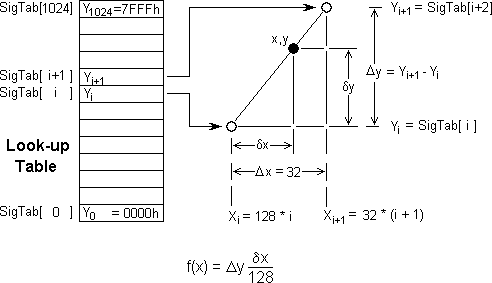The table is chosen to have 1025 entries. This is one more than the range of values 0 to 1023 inclusive since the method needs a final plot beyond the extremity of the range to act as a reference for the last valid plot.

NewVersion of Sigmoid()

To make best use of 16-bit interger precision, this is implemented as a table or ar­ray containing the 1025 values of f(x) which correspond to 1025 values of x which are multiples of 32. Thus x ranges from 0 to 32768 i.e. 0, 32, 64, 96, 128, ... 32736, 32767. The final entry is set to 32767 instead of 32768 to avoid overflow.

So firstly we need a function to create the spot values of f(x) for the table which is stored in the array of short (16-bit) integers SigTab[ ]:

short SigTab;  // values of f(x) for x values which are multiples of 32
void SigGen(void) {
#define R  32767   // maximum extent of both x and f(x)
#define RR 65556   // 65534 + 22
for(int i = 0; i < 1024; i++)
SigTab[i] = (double)(
RR / (1 + exp( -((double)(((long)(i)) << 8)) / R)) - R
);
SigTab = R;
}

The left shift of 8 on the long casting of i multiplies i by 32 to get x and then by 8 to get kx (for k = 8). This function with its time-consuming exp()call need be executed once-only during the network's initialisation phase. So now instead of using the slow exp() function we can find a neurone's output from the sum of its weighted inputs by calling the following function:

int Sigmoid(int x) {
int s, y, j;
if((s = x) < 0) x = -x;   //reverse sign of x if x negative
y = *(SigTab + (j = x >> 5));
y += ((*(SigTab + j + 1) - y) * (x & 0x1F)) >> 5;
if (s < 0) y = -y; //reverse sign of f(x) is x was negative
return(y);
}

This function returns the required value of f(x) in less than 21μs. This is 256 times as fast as the formula. It only disagrees with the formula in the least significant of the 16 bits in only 40% of cases (a maximum error of 30 parts per million). The 256-fold speed gain is merely at the expense of an extra 2k of memory to hold the look-up table. Speeds were measured on an IBM PS/2 Model 30-286 (without an 80287 math co-processor).

Prototyping in QuickBASIC

The thinking behind the Look-up and Interpolation version of Sigmoid() was first validated by prototyping it in Microsoft QuickBASIC. Sigmoid itself was rewritten in QuickBASIC within an exerciser program as follows:

DefInt a - x
dim SigTab(1024)
R!  = 32767
RR! = 65534
screen 9 : color ,1   'switch to EGA enhanced mode graphics
'set up suitable screen co-ordinates
window (-48000,-48000) - (+48000,+48000)
line(-32767, 0) - (32767, 0) , 7
line(0, -32767) - (0, 32767) , 7
locate  2,13
print"NEURAL TRANSFER FUNCTION FOR A MULTI-LAYER PERCEPTRON"
locate  4,39 : print"f(x)"
locate  5,34 : print"+32767"
locate 21,42 : print"-32767"
locate 12,66 : print"+32767"
locate 14,10 : print"-32767"
locate 13,70 : print"x"
locate  8,12 : print"SIGMOID FUNCTION"
locate 16,45 : print"f(x) = 2/(1+exp(-k*x)) - 1"
locate 17,45 : print"x = neuron activation level"
locate 18,45 : print"f(x) = neuron output level"
k =  4 : c = 11 : gosub SigGen : gosub SigTest
k =  8 : c = 10 : gosub SigGen : gosub SigTest
k = 16 : c = 12 : gosub SigGen : gosub SigTest
k = 32 : c = 14 : gosub SigGen : gosub SigTest
LOCATE 24,1:print"HIT ANY KEY TO QUIT";
E: x\$ = inkey\$ : if x\$ = "" goto E
end

SigGen:
for i = 0 to 1023
w! = i
v! = RR! / (1 + exp(-w! * 32 * k / R!)) - R!
y = v! : SigTab(i) = y
next
SigTab(1024) = R!
return

SigTest:
k\$ = str\$(k) : k\$ = "k =" + space\$(3 - len(k\$)) + k\$
locate 11-q,56: print k\$ : q=q+1
x = -32767 : start! = TIMER
' locate 17,5:print"Errors ="
Label:
gosub Sigmoid : pset (x, y), c
if x < 32767 then x = x + 1 : goto Label
locate 23,4:print"TIME:"; TIMER - start! ;"seconds"
' n = nn:l = 22:nn\$ = "Error Occurrences =":gosub ShowNum
return

'THIS IS 1.46 TIMES THE SPEED OF THE EXP() FUNCTION AND
'DISAGREES WITH EXP() ONLY IN BITS 0 & 1 (IE BY AT MOST
'3 IN 32767)

Sigmoid:
if x < 0 then xx = -x else xx = x
xxx! = xx
i = INT(xxx! / 32)
dx = xx - i * 32
y = SigTab(i)
y = y + ((dx * (SigTab(i + 1) - y)) / 32)
if x < 0 then y = -y
' w! = x
' v! = RR! / (1 + exp(-w! * k / R!)) - R!
' yy = v!
' n = abs(yy-y)
' locate 17,13 : print n
' if n > 0 then nn = nn + 1
return

ShowNum:
n\$ = str\$(n) : n\$ = space\$(6 - len(n\$)) + n\$
locate l,5: print nn\$ + n\$
return

This function exercises the look-up table function for 4 different values of k. It can also compare the execution times of the exp and the look-up method and measure the maximum error of the look-up method.

Load sigmoid.bas into a QuickBASIC environment and compile and execute it. This will exercise the QuickBASIC version of the Sigmoid function and display the graph­ical results. You can alter the code in order to experiment with the program.

Always 'comment-out' the display statements in the Sigmoid: subroutine when doing time trials as they are themselves time-consuming. Note that the speed advantage in BASIC between the exp function and the look-up table is nowhere near as much as it is in 'C'.

The 'C' Version

QuickBASIC is a very quick means of getting a prototype like this working and vis­ible. However you cannot use BASIC to optimise efficiency and speed since it does not provide the almost direct control of the CPU that 'C' does. An exerciser for the 'C' version of Sigmoid() was therefore written and exercised within the Microsoft Programmer's Workbench development environment using Microsoft C 6.00 viz:

#include <sys\types.h>
#include <sys\timeb.h>
#include <stdio.h>
#include <graph.h>
#include <math.h>
long PlotErr;               //error-count accumulators

//THE FOLLOWING TWO FUNCTIONS ARE TO BE EXERCISED

short	SigTab;
#define R  32767
#define RR 65556               //65534 + 22

void SigGen(void) {
int i;
for(i = 0; i < 1024; i++)
SigTab[i] = (double)(
RR / (1 + exp( -((double)(((long)(i)) << 8)) / R)) - R
);
SigTab = R;
}

int Sigmoid(int x) {
int s, y, j;
if((s = x) < 0) x = -x;
y = *(SigTab + (j = x >> 5));
y += ((*(SigTab + j + 1) - y) * (x & 0x1F)) >> 5;
if(s < 0) y = -y;
return(y);
}

// BELOW IS THE EXERCISER FOR THE TWO FUNCTIONS ABOVE

#define XYscale 8    //right shift scaling factor
#define Yorg 174     //origin displacement from top left
#define Xorg 171     //origin displacement from top left

void ShowScale(short x1, short y1, short x2, short y2) {
_moveto((x1 >> XYscale) + Xorg, (y1 >> XYscale) + Yorg);
_lineto((x2 >> XYscale) + Xorg, (y2 >> XYscale) + Yorg);
}

double GetTime() {
struct timeb TimeData;
ftime(&TimeData);
return((double)(TimeData.millitm) / 1000 + TimeData.time);
}

main() {
int x, y, X, Y;
double LookTime, ExpTime;
_setvideomode(_VRES16COLOR);
_settextposition(1, 1);
printf("SIGMOID FUNCTION FOR USE IN NEURAL NETWORKS");
_settextposition( 3,19); printf("+32767");
_settextposition(20,19); printf("-32767");
_settextposition(11, 3); printf("-32767");
_settextposition(12,35); printf("+32767");
_setcolor(7);
ShowScale(0, -32767, 0, +32767);  //Draw the x and y axes
ShowScale(-32767, 0, +32767, 0);  //in dull white
_settextposition( 4,46);
printf("The SIGMOID formula is:");
_settextposition( 6,46);
printf("f(x) = (2R+d)/(1+exp(-kx)) - R");
_settextposition( 8,46);
printf("Where R = 32767 and");
_settextposition( 9,46);
printf("-32767 <= x <= +32767");
_settextposition(11,46);
printf("`k' determines how step-like the");
_settextposition(12,46);
printf("function becomes. In this test");
_settextposition(13,46);
printf("k = 8. `k' can conveniently be a");
_settextposition(14,46);
printf("multiple of 2: 2,4,8,16,32,64...");
_settextposition(16,46);
printf("`d' is a small adjusting factor");
_settextposition(17,46);
printf("to make f(x) hit 32767 at exactly");
_settextposition(18,46);
printf("the point at which x hits 32767.");
SigGen();     //generate the look-up table
_setcolor(9); //Draw look-up trace in bright blue
x = -32767;   //Draw the sigmoid curve
Label1:
y = Sigmoid(x);
_setpixel(Xorg + (x >> XYscale), Yorg - (y >> XYscale));
if (x < 32767) { x++; goto Label1; }
_settextposition(24,1);
printf("CHECKING ACCURACY OF LOOKUP & INTERPOLATION...");
_settextposition(6,8); printf("Error");
_setcolor(10);  //Draw formula trace in bright green
x = -32767; Y = 0;
Label2:
if(x < 0) X = -x; else X = x;
y = (double)(
RR / (1 + exp(-((double)(((long)(X)) << 3)) / R)) - R
);
if (x < 0) y = -y;
_setpixel(Xorg + (x >> XYscale), Yorg - (y >> XYscale));
y -= Sigmoid(x);
(PlotErr[y+2])++;  //Increment appropriate error count
if(y > Y) Y = y;
_settextposition(6,14);
printf("%2d", y); //display current error amount
if (x < 32767) { x++; goto Label2; }
_settextposition(7,  8);
printf("MaxErr%2d",Y);
_settextposition(20,43);
printf("RESULTS:");
_settextposition(20,53);
printf("Error = +2:  %6ld",PlotErr);
_settextposition(21,53);
printf("Error = +1:  %6ld",PlotErr);
_settextposition(22,53);
printf("Error =  0:  %6ld",PlotErr);
_settextposition(23,53);
printf("Error = -1:  %6ld",PlotErr);
_settextposition(24,53);
printf("Error = -2:  %6ld",PlotErr);
_settextposition(24,1);
x = -32767;
ExpTime = GetTime();              //Start the timer
Label3:
if(x < 0) X = -x; else X = x;
y = (double)(
RR / (1 + exp(-((double)(((long)(X)) << 3)) / R)) - R
);
if(x < 0) y = -y;
if (x < 32767) { x++; goto Label3; }
ExpTime = GetTime() - ExpTime;    //Stop the timer
x = -32767;
LookTime = GetTime();             //Start the timer
Label4:
y = Sigmoid(x);
if(x < 32767) { x++; goto Label4; }
LookTime = GetTime() - LookTime;  //Stop the timer
_settextposition(26,53);
printf("Exp(x) Time:  %4.2f", ExpTime);
_settextposition(27,53);
printf("Lookup Time:  %4.2f", LookTime);
_settextposition(28,53);
printf("Speed Ratio:  %4.2f", ExpTime/LookTime);
_settextposition(24,1);
printf("FINISHED. HIT `RETURN' TO EXIT.\07");
_settextposition(26,1); getchar();
_setvideomode(_DEFAULTMODE);
}

This source code is contained in the file sigmoid.c and its executable version is in sigmoid.exe. Run sigmoid.exe to exercise the 'C' version of Sigmoid(), or load sigmoid.c into the Microsoft Programmer's Workbench then MAKE and RUN the program. You can modify the code yourself and experiment with it. I later upgraded to Linux where I use the gc compiler.

SigGen( )'s Coding

void SigGen(void) {
#define R  32767     //maximum extent of both x and f(x)
#define RR 65556     //65534 + 22
int i;
for(i = 0; i < 1024; i++)
SigTab[i] = (double)(
RR / (1 + exp( -((double)(((long)(i)) << 8)) / R)) - R
);
SigTab = R;
}

This code is tuned for maximum performance for k = 8. Notice particularly that RR is a little bit more than twice R. In fact it is 22 more than 2 * R. The sigmoid func­tion computed from the exponential formula only reaches its maximum value of 32767 when x reached infinity. To avoid a progressive loss of range across the multi-layer network, x is therefore accentuated slightly so that f(x) hits 32767 precisely when x does.

The value of RR will need to be re-adjusted if you change the value of k. You will have to use something like the following to display the values of x and f(x) so that you can adjust the final few values of f(x) to intercept the maximum value of 32767 smoothly:

main() {
#define R  32767   //maximum extent of both x and f(x)
#define RR 65556   //65534 + 22
#define K      8   //set the value of K you want here
int x;
for(x = 0; x < 32766; x++) {
y = (double)(
RR / (1 + exp( -(((double)(x)) * K) / R)) - R
);
printf("x = %5d     f(x) = %5d\n", x, y);
}
}

See source file siggen.c and executable siggen.exe.

Sigmoid( )'s Coding

int Sigmoid(int x) {
int s, y, j;
if ((s = x) < 0) x = -x;   //reverse sign of x if negative
y = *(SigTab + (j = x >> 5));
y += ((*(SigTab + j + 1) - y) * (x & 0x1F)) >> 5;
if(s < 0) y = -y; //reverse sign of f(x) if x was negative
return(y);
}

Because of its time-critical position at the very centre of the innermost loop of the Multi-layer Perceptron neural network function MLP(), great effort has been made to make Sigmoid() as fast as possible.

Thus multiplications and divisions have been replaced wherever possible by binary shifts, and remainder extraction has been replaced by logical masking. This has been implemented in Sigmoid() as shown below: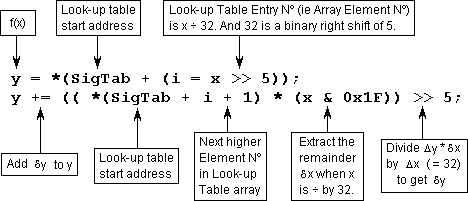Because the above two statements will work only with positive numbers, negative values are reversed before and after the two above statements. Testing for less than zero was found to be the quickest and safest way to reverse the signs of neg­ative values since the 80x86 CPU represents negative values as the complement of the equivalent positive values:

+32767     7FFF
+    1     0001
-    1     FFFF
-32767     8001

This does not make it easy to change the sign by bitwise logic. However, if you are using a CPU with 'sign-mantissa' arithmetic, you could improve the speed of Sigmoid() even further by coding it as follows:

int Sigmoid(int s) {
int x, y, j;
s = x & 0x7FFF;             //reverse sign of x if negative
y = *(SigTab + (j = x >> 5));
return(
(s & 0x8000) |
(y += ((*(SigTab + j + 1) - y) * (x & 0x1F)) >> 5)
);
}

This won't work on machines that use complement arithmetic and masks must be compatible with the machine's word-length. A 16-bit word length is assumed ab­ove. The function becomes quite machine-dependent, but the extra speed could be worth it if you do a lot of network training runs.

Test Results

The following table shows the results produced by Sigmoid() while running in the above exerciser. It shows how many of the 65335 values of f(x) returned by Sigmoid() were in error by +2, +1, 0, −1, −2 compared with the corresponding value returned by the exp(x) formula: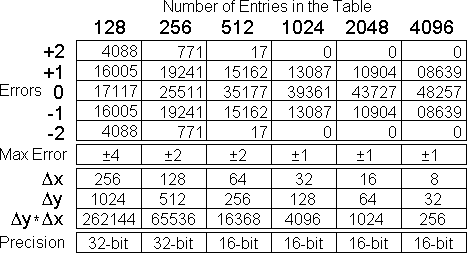As can be seen from the lower part of the above table, a long casting of Dy had to be used for tables with 256 entries or less otherwise the product Dy * Dx would overflow the 16-bit short register. For a 256-entry table, the 4th line of Sigmoid() therefore had to become:

y += ((long)((*(SigTab + i + 1) − y)) * (x & 0x7F)) >> 7;

This however resulted in a 20% degradation in speed as shown by the following time trial results: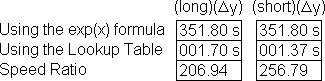Because it helped speed up the BASIC version, an attempt to preserve as much speed as possible was made by switching precision based on the value of dx as follows:

short Sigmoid(short s) {
int x, y, i, dx, Dy;   //dx represents dx, Dy represents Dy
if((x = s) < 0) x = -x;
y = *(SigTab + (i = x >> 7));
if((dx = x & 0x7F) > 0) {       //if we need to interpolate
Dy = *(SigTab + i + 1) - y;   //diff between two y entries
if (Dy < 259)
y += (Dy * dx) >> 7;            //use 16-bit precision
else
y += ((long)(Dy)) * dx) >> 7;   //use 32-bit precision
}
if (s < 0) y = -y;
return(y);
}

This however had the opposite effect due to the time taken up by the extra if statements.

The optimum size of look-up table was therefore 1024 giving a firm value of f(x) for increments in x of 32 with a maximum error of ±1. Nothing was gained by using larger tables since the error in the least significant bit would be present anyway from binary rounding errors on the shifts and multiplies. Besides this is only an error of 30 parts per million which is better than the inputs originating from most input sources. Smaller tables started to allow the linear interpolation errors to creep in rather rapidly.

Other Transfer Functions

The sigmoid is the ideal non-linear transfer function for the multi-layer perceptron. However, there are other kinds of neural network topologies in which different non-linear transfer functions work better. For instance, in the Radial Basis Network top­ology, the Gaussian (or bell curve) function is used. This function, together with a test exerciser are available in gauss.c (executable in gauss.exe) and gauss_test.c (executable in gauss_test.exe).

Sigmoid Generator Applet

Generates either:
• a unipolar sigmoid in which both x and y range from 0 to +1, or
• a bipolar sigmoid in which both x and y range from -1 to +1.

Which type of sigmoid is produced is determined by the content of the 'param' tag within the 'applet' tag used to invoke the applet.

The code of this applet could easily be extended to include the generation of the look-up table previously done by the 'C' program described in the text.

/**
* Sigmoid Function Generator
* @author Robert John Morton
* @version 15 December 1997
*/

import java.awt.*;

public class Sigmoid extends java.applet.Applet implements Runnable
{
boolean finished = false;   //indicates when the curve has been completed
double k = .025;            //non-linearity constant
double y = k / 4;           //output value
double x = 0;               //input value
double dx = k / 10;         //plotting (or look-up table) increment
int s = 200;                //pixels per unit real (scaling factor)
int H = 0, V = s - 1;       //window co-ordinate of graphical origin
int X, Y;                   //dimensions (in pixels) of the applet window
Image I = null;             //reference for an off-screen image I
Graphics i = null;          //graphics context for the off-screen image
long TF = 50;               //total Time Frame for plotting update cycle
long T;                     //time at which next new cycle is due to begin
Font font;                  //and a font reference for annotations
Color tr;                   //trace colour
boolean TypeFlag = false;   //set for unipolar presentation

public void init() {              //INITIALISE THE APPLET
int Xy, Yx;                    //co-ords of axis labels
tr = new Color( 0, 128, 64);   //create special dark green for trace

// lettering font for this applet
font = new Font("Dialog", Font.PLAIN, 12);

Dimension d = size();   //get size of window as given in HTML applet tag

X = d.width; Y = d.height;     //establish window width and height
I = createImage(X, Y);         //create the off-screen image I

i = I.getGraphics();   //graphics context reference for off-screen image

/* If applet parameter 'type' = 'bipolar' then set the TypeFlag true,
the non-linearity constant, the output value, the input value, the
plotting (or look-up table) increment, the number of pixels per unit
real (scaling factor), the window co-ordinate of the graphics origin
and the co-ords of the axis letters. */

if(getParameter("type").equals("bipolar")){
TypeFlag = true; k = .011; y = 0; x = 0; dx = .0025; s = 100;
H = 100; V = 100; Xy = V + 10; Yx = H - 10;
}

/* Else, if doing a unipolar graph,
just set the co-ords of the axis letters. */

else { Xy = V - 5; Yx = H + 5; }

i.setColor(Color.lightGray);   //background colour for off-screen image
i.fillRect(0, 0, X, Y);        //paint background of off-screen image
i.setFont(font);               //set up the annotation font
i.setColor(Color.gray);        //set colour for drawing the graph axes
i.drawLine(0, V, X, V);        //draw x axis
i.drawLine(H, 0, H, Y);        //draw y axis
i.setColor(Color.black);       //set colour for lettering

i.drawString("X", X - 10, Xy);
i.drawString("Y", Yx, 10);     //print the X and Y axis letters
i.setColor(tr);                //set colour to paint trace on image

T = System.currentTimeMillis() + TF;   //end time for current time frame
}

public void paint(Graphics g) {      //set up the graphics
g.drawImage(I, 0, 0, null);       //(re)draw from the off-screen image I
}

public void update(Graphics g) {     //PLOT THE SIGMOID GRAPH
if(x > 1 || y > 1)          //if plot has reached edge of graph
finished = true;               //set the 'finished' flag
else {                            //if not yet finished plotting graph
int h = (int)(s * x);          //convert horizontal plot to pixels
int v = (int)(s * y);          //convert vertical plot to pixels
g.setColor(tr);                //set colour to paint trace on screen
int a = h, b, c = V - v, d;    //simplify pixel co-ordinates
if(TypeFlag) {
a = H - h; b = H + h;       //simplify pixel co-ordinates
c = V + v; d = V - v;
g.drawLine(b, d, b, d);     //do next plot in lower left quadrant
i.drawLine(b, d, b, d);     //do next plot in lower left quadrant
y += k * (1 - y);           //advance output difference equation
} else
y += k * y * (1 - y);       //advance output difference equation
x += dx;                       //advance the input value
g.drawLine(a, c, a, c);        //do next plot in upper right quadrant
i.drawLine(a, c, a, c);        //do next plot in upper right quadrant
}
}

public void run() {              //run the plotting thread
while(true) {                 //permanent loop broken by external event
if(!finished) repaint();   //if not yet finished, do next plot

/* Get time remaining in this cycle's time frame, setting a lower limit
in case the host PC is too slow for the ideal trace speed. */

long s = T - System.currentTimeMillis(); if (s < 5) s = 5;

/* Try to sleep for the time remaining in the current time frame, while
allowing browser events to interrupt the thread. This happens if you
return to applet's HTML page. Then set the finish time of the next
time frame. */

} catch (InterruptedException e) { }
T = System.currentTimeMillis() + TF;
}
}

public void start() {             //Start program thread by